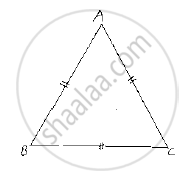# Prove that Each Angle of an Equilateral Triangle is 60°. - Mathematics

Prove that each angle of an equilateral triangle is 60°.

#### Solution

Given to prove that each angle of an equilateral triangle is 60°
Let us consider an equilateral triangle ABC
Such that AB= BC= CA

Now,

AB=BC ⇒ ∠A=∠C            ................(1) [Opposite angles to equal sides are equal]

and BC = AC ⇒∠B = ∠A ……..(2)

From (1) and (2), we get

∠A = ∠B = ∠C          ..............(3)

We know that
Sum of angles in a triangle =180°

⇒∠A+∠B+∠C=180°

⇒ ∠A+∠A+∠A=180°

⇒3 ∠A=180°

⇒ ∠A=(180°)/3=60°

∴∠S=∠B=∠C=60°

Hence, each angle of an equilateral triangle is 60°.Concept: Properties of a Triangle
Is there an error in this question or solution?

#### APPEARS IN

RD Sharma Mathematics for Class 9
Chapter 12 Congruent Triangles
Exercise 12.3 | Q 4 | Page 47

Share